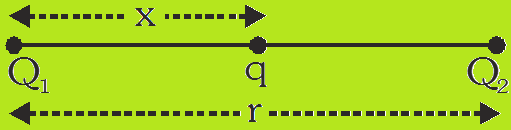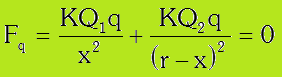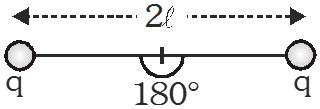# Electrostatic Equilibrium of charged particles

Electrostatic Equilibrium:- In equilibrium net electric force on every charged particle is zero. The equilibrium of a charged particle, under the action of Colombian forces alone can never be stable.

Equilibrium is of three types:-

(a) Stable Equilibrium : A charge is initially in equilibrium position and is displaced by a small distance. If the charge tries to return back to the same equilibrium position then this equilibrium is called position of stable equilibrium.

(b) Unstable Equilibrium : If charge is displaced by a small distance from its equilibrium position and the charge has no tendency to return to the same equilibrium position. Instead it goes away from the equilibrium position.

(b) Neutral Equilibrium : If charge is displaced by a small distance and it is still in equilibrium condition then it is called neutral equilibrium.

Problem Solving strategies:-

(1) Equilibrium of three point charges:-(i) Two charges must be of like nature as(ii) Third charge should be of unlike nature asTherefore(2) Equilibrium of symmetric geometrical point charged system:-

Value of Q at centre for which system to be in state of equilibrium:-

Q=[ -q/√3 ]

Q= [ -q(2√2+1)/4 ]

(3) Equilibrium of suspended point charge system:-T cosθ = mg  and  T sinθ = Fe = kQ2/x

⇒ tanθ = [ Fe /mg ] = [kQ2/x2mg ]

If q is small then

tanθ ≅ sinθ = [x/2l]    ⇒    x/2l = kQ2/x2mg

⇒ x3 = 2kQ2l/mg

⇒ x = [ Q2l/2πε0mg ]1/3

If whole set up is taken into an artificial satellite (g≅0) :-then  T = Fe = kq2/4l2